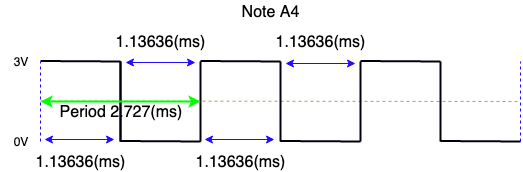The micro:bit uses Pulse Width Modulation (PWM) as a way to simulate an analogue output on a digital pin. It sends a series of high speed on/off electronic pulses to a speaker which can convert this to physical vibrations to create sound waves.

The variation in the length of the on pulse, the Duty Cycle creates an average voltage output. A 50% duty cycle (often called a square wave) sets an equal time for pulse on and off.To convey the frequency of sound(Hz) that the digital signal is trying to reproduce we vary the time between the signal voltage being high or low.

To do this,  the period(ms) of the note needs to be found, which is the amount of time it takes for the wave to cycle once. This is done by taking the inverse of the frequency of the note:

Period(ms)=1/Frequency(Hz)

For example, The note A4 maps to 440hz so using our calculation:

1/440 = 2.727

Once we have the value of the period, to represent the pitch in PWM, we just hold the signal high for half the length of the period (1.13636ms), and low for the other half(1.13636ms)The Analogue Pitch block in MakeCode lets us set this frequency for PWM on the desired pin.